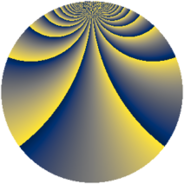# Properties

 Label 966.2.bdLevel $966$ Weight $2$ Character orbit 966.bd Rep. character $\chi_{966}(59,\cdot)$ Character field $\Q(\zeta_{66})$ Dimension $1280$ Newform subspaces $1$ Sturm bound $384$ Trace bound $0$

# Related objects

## Defining parameters

 Level: $$N$$ $$=$$ $$966 = 2 \cdot 3 \cdot 7 \cdot 23$$ Weight: $$k$$ $$=$$ $$2$$ Character orbit: $$[\chi]$$ $$=$$ 966.bd (of order $$66$$ and degree $$20$$) Character conductor: $$\operatorname{cond}(\chi)$$ $$=$$ $$483$$ Character field: $$\Q(\zeta_{66})$$ Newform subspaces: $$1$$ Sturm bound: $$384$$ Trace bound: $$0$$

## Dimensions

The following table gives the dimensions of various subspaces of $$M_{2}(966, [\chi])$$.

Total New Old
Modular forms 4000 1280 2720
Cusp forms 3680 1280 2400
Eisenstein series 320 0 320

## Trace form

 $$1280q - 64q^{4} + 4q^{9} + O(q^{10})$$ $$1280q - 64q^{4} + 4q^{9} + 16q^{15} + 64q^{16} - 44q^{18} + 120q^{21} - 16q^{22} - 12q^{24} + 56q^{25} + 32q^{30} - 24q^{33} + 8q^{36} - 44q^{37} - 20q^{39} + 4q^{42} + 136q^{43} + 12q^{45} + 12q^{46} + 92q^{49} + 4q^{51} - 36q^{54} - 56q^{57} - 28q^{58} + 8q^{60} + 72q^{61} - 134q^{63} + 128q^{64} + 24q^{67} - 72q^{70} - 44q^{72} - 72q^{73} + 48q^{75} - 16q^{78} - 72q^{79} + 40q^{81} + 48q^{82} - 10q^{84} - 32q^{85} + 222q^{87} - 8q^{88} - 8q^{91} - 16q^{93} + 72q^{94} - 12q^{96} - 68q^{99} + O(q^{100})$$

## Decomposition of $$S_{2}^{\mathrm{new}}(966, [\chi])$$ into newform subspaces

Label Dim. $$A$$ Field CM Traces $q$-expansion
$$a_2$$ $$a_3$$ $$a_5$$ $$a_7$$
966.2.bd.a $$1280$$ $$7.714$$ None $$0$$ $$0$$ $$0$$ $$0$$

## Decomposition of $$S_{2}^{\mathrm{old}}(966, [\chi])$$ into lower level spaces

$$S_{2}^{\mathrm{old}}(966, [\chi]) \cong$$ $$S_{2}^{\mathrm{new}}(483, [\chi])$$$$^{\oplus 2}$$